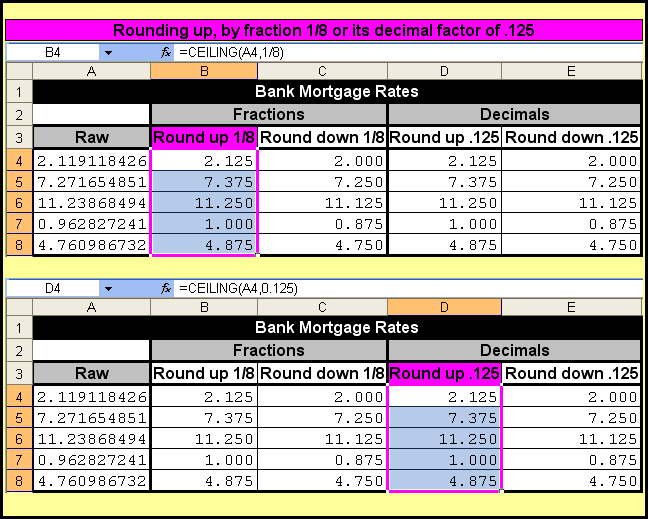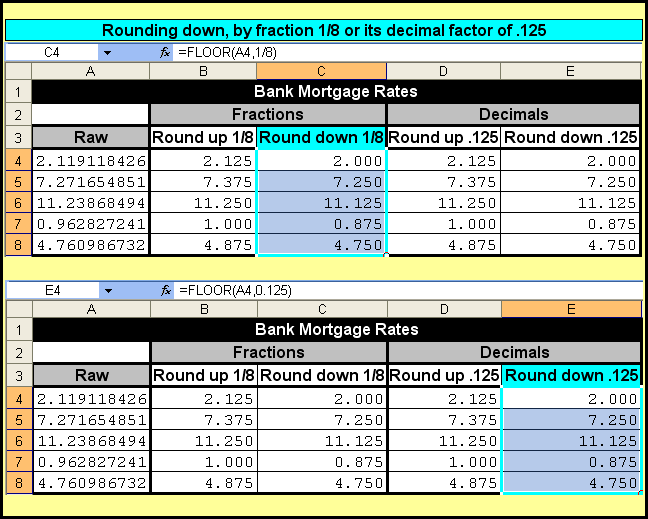Rounding Numbers By Fractions or Decimals - KING OF EXCEL

## Saturday, December 21, 2019

Rounding Numbers By Fractions or Decimals
When rounding numbers to a particular decimal factor, you can express that rounding factor in your formula as either a fraction or as its decimal equivalent. In the pictures, the fraction one-eighth can be stated in a formula as 1/8 or by its decimal equivalent of .125.
Rounding up
Expressing a fraction, the formula in cell B4 copied to cell B8 is `=CEILING(A4,1/8)`
Expressing a decimal, the formula in cell D4 copied to cell D8 is `=CEILING(A4,0.125)`
Notice the results in range B4:B8 are the same as the results in range D4:D8.Rounding down
Expressing a fraction, the formula in cell C4 copied to cell C8 is `=FLOOR(A4,1/8)`
Expressing a decimal, the formula in cell E4 copied to cell E8 is `=FLOOR(A4,0.125)`
Notice the results in range C4:C8 are the same as the results in range E4:E8.#evba #etipfree
📤You download App EVBA.info installed directly on the latest phone here : https://www.evba.info/p/app-evbainfo-setting-for-your-phone.html?m=1